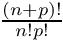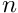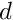GetFEM  5.4.2
bgeot Namespace Reference

Basic Geometric Tools. More...

## Classes

class  Comma_initializer
Template class which forwards insertions to the container class. More...

class  convex
generic definition of a convex ( bgeot::convex_structure + vertices coordinates ) More...

class  convex_of_reference
Base class for reference convexes. More...

class  convex_structure
Structure of a convex. More...

class  geometric_trans
Description of a geometric transformation between a reference element and a real element. More...

class  geotrans_interpolation_context
the geotrans_interpolation_context structure is passed as the argument of geometric transformation interpolation functions. More...

class  geotrans_inv
handles the geometric inversion for a given (supposedly quite large) set of points More...

class  geotrans_inv_convex
does the inversion of the geometric transformation for a given convex More...

class  geotrans_precomp_
precomputed geometric transformation operations use this for repetitive evaluation of a geometric transformations on a set of points "pspt" in the reference convex which do not change. More...

class  geotrans_precomp_pool
The object geotrans_precomp_pool Allow to allocate a certain number of geotrans_precomp and automatically delete them when it is deleted itself. More...

struct  imbricated_box_less
A comparison function for bgeot::base_node. More...

struct  index_node_pair
store a point and the associated index for the kdtree. More...

class  kdtree
Balanced tree over a set of points. More...

class  mesh_structure
Mesh structure definition. More...

class  node_tab
Store a set of points, identifying points that are nearer than a certain very small distance. More...

class  permutation
generation of permutations, and ranking/unranking of these. More...

class  polynomial
This class deals with plain polynomials with several variables. More...

class  power_index
Vector of integer (16 bits type) which represent the powers of a monomial. More...

class  rtree
Balanced tree of n-dimensional rectangles. More...

class  small_vector
container for small vectors of POD (Plain Old Data) types. More...

struct  stored_point_tab
Point tab storage. More...

struct  torus_geom_trans

class  torus_structure
torus_structure which extends a 2 dimensional structure with a radial dimension More...

## Typedefs

typedef gmm::uint16_type short_type
used as the common short type integer in the library

typedef std::shared_ptr< const convex_structurepconvex_structure
Pointer on a convex structure description.

typedef std::shared_ptr< const bgeot::geometric_transpgeometric_trans
pointer type for a geometric transformation

typedef std::vector< index_node_pairkdtree_tab_type
store a set of points with associated indexes.

typedef size_t size_type
used as the common size type in the library

## Functions

pconvex_ref simplex_of_reference (dim_type nc, short_type k=1)
returns a simplex of reference of dimension nc and degree k

pconvex_ref Q2_incomplete_of_reference (dim_type d)
incomplete Q2 quadrilateral/hexahedral of reference of dimension d = 2 or 3

pconvex_ref pyramid_QK_of_reference (dim_type k)
pyramidal element of reference of degree k (k = 1 or 2 only)

pconvex_ref pyramid_Q2_incomplete_of_reference ()
incomplete quadratic pyramidal element of reference (13-node)

pconvex_ref prism_incomplete_P2_of_reference ()
incomplete quadratic prism element of reference (15-node)

pconvex_ref convex_ref_product (pconvex_ref a, pconvex_ref b)
tensorial product of two convex ref. More...

pconvex_ref parallelepiped_of_reference (dim_type nc, dim_type k=1)
parallelepiped of reference of dimension nc (and degree 1)

pconvex_ref prism_of_reference (dim_type nc)
prism of reference of dimension nc (and degree 1)

pconvex_ref equilateral_simplex_of_reference (dim_type nc)
equilateral simplex (degree 1). More...

pconvex_ref generic_dummy_convex_ref (dim_type nc, size_type n, short_type nf)
generic convex with n global nodes

bool operator== (const pconvex_structure &p1, const pconvex_structure &p2)
Stored objects must be compared by keys, because there is a possibility that they are duplicated in storages of multiple threads and pointers to them are never equal.

bool operator== (const pconvex_structure &p1, std::nullptr_t)
these operators still use comparison by addresses against nullptr

int get_token (std::istream &ist, std::string &st, bool ignore_cr=true, bool to_up=true, bool read_un_pm=true, int *linenb=0)
Very simple lexical analysis of general interest for reading small languages with a "MATLAB like" syntax : spaces are ignored, '' indicates a commentary until the end of the line, '...' indicates that the instruction continue on the next line (or separate two sub part of the same character string). More...

void cuthill_mckee_on_convexes (const bgeot::mesh_structure &ms, std::vector< size_type > &cmk)
Return the cuthill_mc_kee ordering on the convexes.

size_type alpha (short_type n, short_type d)
Return the value ofwhich is the number of monomials of a polynomial ofvariables and degree.

base_poly read_base_poly (short_type n, std::istream &f)
read a base_poly on the stream ist.

base_poly read_base_poly (short_type n, const std::string &s)
read a base_poly on the string s.

void structured_mesh_for_convex (pconvex_ref cvr, short_type k, pbasic_mesh &pm, pmesh_precomposite &pmp, bool force_simplexification)
This function returns a mesh in pm which contains a refinement of the convex cvr if force_simplexification is false, refined convexes have the same basic_structure than cvr, if it is set to true, the cvr is decomposed into simplexes which are then refined. More...

const basic_mesh * refined_simplex_mesh_for_convex (pconvex_ref cvr, short_type k)
simplexify a convex_ref. More...

const std::vector< std::unique_ptr< mesh_structure > > & refined_simplex_mesh_for_convex_faces (pconvex_ref cvr, short_type k)
simplexify the faces of a convex_ref More...

pconvex_ref basic_convex_ref (pconvex_ref cvr)
return the associated order 1 reference convex.

pconvex_structure basic_structure (pconvex_structure cv)
Original structure (if concerned)

template<typename T >
std::ostream & operator<< (std::ostream &o, const polynomial< T > &P)
Print P to the output stream o. for instance cout << P;.

template<typename T >
polynomial< T > poly_substitute_var (const polynomial< T > &P, const polynomial< T > &S, size_type subs_dim)
polynomial variable substitution More...

template<typename T >
rational_fraction< T > operator+ (const polynomial< T > &P, const rational_fraction< T > &Q)

template<typename T >
rational_fraction< T > operator- (const polynomial< T > &P, const rational_fraction< T > &Q)
Subtract Q from P.

## functions on geometric transformations

typedef std::shared_ptr< const geotrans_precomp_pgeotrans_precomp

typedef dal::naming_system< geometric_trans >::param_list gt_param_list

void mat_mult (const scalar_type *A, const scalar_type *B, scalar_type *C, size_type M, size_type N, size_type P)

void mat_tmult (const scalar_type *A, const scalar_type *B, scalar_type *C, size_type M, size_type N, size_type P)

scalar_type lu_det (const scalar_type *A, size_type N)

scalar_type lu_inverse (scalar_type *A, size_type N, bool doassert)

pgeometric_trans Q2_incomplete_geotrans (dim_type nc)

pgeometric_trans pyramid_QK_geotrans (short_type k)

pgeometric_trans pyramid_Q2_incomplete_geotrans ()

pgeometric_trans prism_incomplete_P2_geotrans ()

base_small_vector compute_normal (const geotrans_interpolation_context &c, size_type face)
norm of returned vector is the ratio between the face surface on the real element and the face surface on the reference element IT IS NOT UNITARY More...

base_matrix compute_local_basis (const geotrans_interpolation_context &c, size_type face)
return the local basis (i.e. More...

void add_geometric_trans_name (std::string name, dal::naming_system< geometric_trans >::pfunction f)

pgeometric_trans geometric_trans_descriptor (std::string name)
Get the geometric transformation from its string name. More...

std::string name_of_geometric_trans (pgeometric_trans p)
Get the string name of a geometric transformation. More...

pgeometric_trans simplex_geotrans (size_type n, short_type k)

pgeometric_trans parallelepiped_geotrans (size_type n, short_type k)

pgeometric_trans parallelepiped_linear_geotrans (size_type n)

pgeometric_trans prism_linear_geotrans (size_type n)

pgeometric_trans linear_product_geotrans (pgeometric_trans pg1, pgeometric_trans pg2)

pgeometric_trans prism_geotrans (size_type n, short_type k)

pgeometric_trans product_geotrans (pgeometric_trans pg1, pgeometric_trans pg2)

pgeometric_trans default_trans_of_cvs (pconvex_structure cvs)

pgeotrans_precomp geotrans_precomp (pgeometric_trans pg, pstored_point_tab pspt, dal::pstatic_stored_object dep)

void delete_geotrans_precomp (pgeotrans_precomp pgp)

IS_DEPRECATED pgeometric_trans APIDECL pyramid_geotrans (short_type k)

scalar_type lu_det (const base_matrix &A)

scalar_type lu_inverse (base_matrix &A, bool doassert=true)

## Detailed Description

Basic Geometric Tools.

## ◆ convex_ref_product()

 pconvex_ref bgeot::convex_ref_product ( pconvex_ref a, pconvex_ref b )

tensorial product of two convex ref.

in order to ensure unicity, it is required the a->dim() >= b->dim()

Definition at line 729 of file bgeot_convex_ref.cc.

## ◆ equilateral_simplex_of_reference()

 pconvex_ref bgeot::equilateral_simplex_of_reference ( dim_type nc )

equilateral simplex (degree 1).

used only for mesh quality estimations

Definition at line 831 of file bgeot_convex_ref.cc.

## ◆ operator<<()

 std::ostream & bgeot::operator<< ( std::ostream & o, const convex_structure & cv )

Print the details of the convex structure cvs to the output stream o.

For debuging purpose.

Definition at line 71 of file bgeot_convex_structure.cc.

## ◆ simplex_structure()

 pconvex_structure bgeot::simplex_structure ( dim_type n, short_type k )

Simplex structure with the Lagrange grid of degree k.

Parameters
 n the simplex dimension. k the simplex degree.

Definition at line 238 of file bgeot_convex_structure.cc.

## ◆ get_token()

 int bgeot::get_token ( std::istream & ist, std::string & st, bool ignore_cr = true, bool to_up = true, bool read_un_pm = true, int * linenb = 0 )

Very simple lexical analysis of general interest for reading small languages with a "MATLAB like" syntax : spaces are ignored, '' indicates a commentary until the end of the line, '...' indicates that the instruction continue on the next line (or separate two sub part of the same character string).

The function returns 0 if there is nothing else to read in the file 1 if an end line has been found (st is empty in this case) 2 if a number as been read 3 if a string has been read 4 if a alphanumeric name has been read 5 for a one character symbol 6 for a two characters symbol (<=, >=, ==, !=, &&, ||)

Note that when the end of line is not significant the option ignore_cr allows to consider the carriage return as a space character.

Definition at line 50 of file bgeot_ftool.cc.

## ◆ compute_normal()

 base_small_vector APIDECL bgeot::compute_normal ( const geotrans_interpolation_context & c, size_type face )

norm of returned vector is the ratio between the face surface on the real element and the face surface on the reference element IT IS NOT UNITARY

pt is the position of the evaluation point on the reference element

Definition at line 1082 of file bgeot_geometric_trans.cc.

## ◆ compute_local_basis()

 base_matrix APIDECL bgeot::compute_local_basis ( const geotrans_interpolation_context & c, size_type face )

return the local basis (i.e.

the normal in the first column, and the tangent vectors in the other columns

Definition at line 1094 of file bgeot_geometric_trans.cc.

## ◆ geometric_trans_descriptor()

 pgeometric_trans APIDECL bgeot::geometric_trans_descriptor ( std::string name )

Get the geometric transformation from its string name.

name_of_geometric_trans

Definition at line 1163 of file bgeot_geometric_trans.cc.

## ◆ name_of_geometric_trans()

 std::string APIDECL bgeot::name_of_geometric_trans ( pgeometric_trans p )

Get the string name of a geometric transformation.

List of possible names: GT_PK(N,K) : Transformation on simplexes, dim N, degree K

GT_QK(N,K) : Transformation on parallelepipeds, dim N, degree K GT_PRISM(N,K) : Transformation on prisms, dim N, degree K GT_PYRAMID_QK(K) : Transformation on pyramids, dim 3, degree K=0,1,2 GT_Q2_INCOMPLETE(N) : Q2 incomplete transformation in dim N=2 or 3. GT_PYRAMID_Q2_INCOMPLETE : incomplete quadratic pyramid transformation in dim 3 GT_PRISM_INCOMPLETE_P2 : incomplete quadratic prism transformation in dim 3 GT_PRODUCT(a,b) : tensorial product of two transformations GT_LINEAR_PRODUCT(a,b) : Linear tensorial product of two transformations GT_LINEAR_QK(N) : shortcut for GT_LINEAR_PRODUCT(GT_LINEAR_QK(N-1), GT_PK(1,1))

Definition at line 1173 of file bgeot_geometric_trans.cc.

## ◆ structured_mesh_for_convex()

 void bgeot::structured_mesh_for_convex ( pconvex_ref cvr, short_type k, pbasic_mesh & pm, pmesh_precomposite & pmp, bool force_simplexification )

This function returns a mesh in pm which contains a refinement of the convex cvr if force_simplexification is false, refined convexes have the same basic_structure than cvr, if it is set to true, the cvr is decomposed into simplexes which are then refined.

TODO: move it into another file and separate the pmesh_precomposite part ?

Definition at line 499 of file bgeot_poly_composite.cc.

## ◆ refined_simplex_mesh_for_convex()

 const basic_mesh * bgeot::refined_simplex_mesh_for_convex ( pconvex_ref cvr, short_type k )

simplexify a convex_ref.

Parameters
 cvr the convex_ref. k the refinement level.
Returns
a pointer to a statically allocated mesh. Do no free it!

Definition at line 558 of file bgeot_poly_composite.cc.

## ◆ refined_simplex_mesh_for_convex_faces()

 const std::vector< std::unique_ptr< mesh_structure > > & bgeot::refined_simplex_mesh_for_convex_faces ( pconvex_ref cvr, short_type k )

simplexify the faces of a convex_ref

Parameters
 cvr the convex_ref. k the refinement level.
Returns
vector of pointers to a statically allocated mesh_structure objects. Do no free them! The point numbers in the mesh_structure refer to the points of the mesh given by refined_simplex_mesh_for_convex.

Definition at line 565 of file bgeot_poly_composite.cc.

## ◆ prism_P1_structure()

 pconvex_structure bgeot::prism_P1_structure ( dim_type nc )
inline

Give a pointer on the structures of a prism of dimension d.

i.e. the direct product of a simplex of dimension d-1 and a segment.

Definition at line 206 of file bgeot_convex_structure.h.

## ◆ poly_substitute_var()

template<typename T >
 polynomial bgeot::poly_substitute_var ( const polynomial< T > & P, const polynomial< T > & S, size_type subs_dim )

polynomial variable substitution

Parameters
 P the original polynomial S the substitution poly (not a multivariate one) subs_dim : which variable is substituted example: poly_subs(x+y*x^2, x+1, 0) = x+1 + y*(x+1)^2

Definition at line 587 of file bgeot_poly.h.